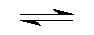## Hexa Aqua Sodium(I+) and Calcium(II+) Cation  Complex Hydrolise by Aris Kaksis Department of medical Biochemistry, RigaStradin University RSU

At equilibrium, the following equilibrium equation is established with its conjugate base:
Na+(H2O)6 (aq)      +      H2O (l)Na+(H2O)5(OH-) (aq)   + H3O+ (aq)
acid extremely weak                                                                 conjugate strong base
The acid and its conjugate base have different colours. At low pH values the concentration of H3O+ is high and so
the equilibrium position lies to the left. The equilibrium solution has the colour A. At high pH values,the concentration
of H3O+is low - the equilibrium position thus lies to the right and the equilibrium solution has colour B.

NaOH5HOHacdSM.skc
NaOH5HOHacdSM.mol      NaOH5HOHacdSM.tgf

blue (Acid)        pHhydrolize=14.2               red (Base)

We can apply equilibrium law to Brønsted equilibria - in general for a weak acid sodium complex:
Keq = ( [H3O+]·[ Na+(H2O)5(OH-)] / ([Na+(H2O)6]•[ H2O]) ) eq
Keq is known as the Brønsted equilibria constant. The pH for hydrolyse is calculated at this point when:
[ Na+(H2O)5(OH-)]   =   [Na+(H2O)6]
So from equation: Keq = [H3O+] / [ H2O] =10-14.2/ [ H2O] = 10-14.2/ 55.3 = 10-15.9
Keq = [H3O+] / [H2O]; Keq•[H2O] = [H3O+] =10-14.2; -log(Keq•[H2O]) = 14.2 = pH = -log([H3O+])
The pH of the solution at its equilibrium point is called the pH and is the pH at which half of the sodium is in its
acid form and the other half in the form of its conjugate base.

At a low pH<14.2, a weak acid aqua complex of sodium ion is almost entirely in the [Na+(H2O)6] form, the
concentration of which predominates. As the pH increases pH>14.2 - the concentration of [Na+(H2O)6] decreases
and the equilibrium is pushed to the right to conjugate base [ Na+(H2O)5(OH-)] form, the concentration of which predominates.

Aqua complex of sodium

We can apply equilibrium law to complexe stability constant equilibria - in general for a acid form sodium cation:

Na+(aq)      +   OH- (aqNa+(OH-)(aq)
blue (Acid)                                                             red (Base)
Kstab=[ Na+(OH-)]/([Na+]•[ OH-]) =10-0.2= ([ Na+(OH-)][H3O+]) / ([Na+]•[10-14])
Kstab is known as the Complexe stability constant. The pH for hydrolyse is calculated at this point when:

[ Na+(OH-)]   =   [Na+]
So from equation: Kstab = [H3O+] / 10-14 =10-0.2
Kstab•10-14 = [H3O+] =10 -14.2 ;  -log(Kstab•10-14) = 14.2 = pH = -log([H3O+])
The pH of the solution at its equilibrium point is called the pH and is the pH at which half of the sodium is in its
conjugate base and the other half in the form of its acid form.

Complex maker of sodium cation
At a low pH<14.2 a weak acid aqua complex of maganesium ion is almost entirely in the Na+ form free cation, the
concentration of which predominates. As the pH increases pH>14.2 - the concentration of [Na+] decreases and the
equilibrium is pushed to the right to conjugate base    [Na+(OH-)] form, the concentration of which predominates.

Aqua complex of calcium

At equilibrium, the following equilibrium equation is established with its conjugate base:
Ca2+(H2O)6 (aq)      +      H2O (l)Ca2+(H2O)5(OH-) (aq)   + H3O+ (aq)
acid extremely weak                                   conjugate strong base
The acid and its conjugate base have different colours. At  pH=14.2 values the concentration of H3O+ is low as
basic medium and so the equilibrium position lies on the high pH numbers. The equilibrium solution has
the colour red. pH<14.2 blue (Acid)     midle pH=14.2                     pH>14.2 red (Base)

Literature

Handbook of Chemical Equilibria in Analytical Chemistry. S.Kortly, L.Sucha. New York, Ellis Horwood LTD.1985.414.p.

We can apply equilibrium law to Brønsted equilibria - in general for a weak acid calcium complex:
Keq = ( [H3O+]·[ Ca2+(H2O)5(OH-)] / ([Ca2+(H2O)6]•[ H2O]) ) eq
Keq is known as the Brønsted equilibria constant. The pH for hydrolyse is calculated at this point
when:                    [ Ca2+(H2O)5(OH-)]   =   [Ca2+(H2O)6]
So from equation: Keq = [H3O+] / [H2O] =10-12.85/ [ H2O] = 10-12.85/ 55.3 = 10-14.59
Keq = [H3O+] / [H2O]; Keq•[H2O] = [H3O+] =10-12.85; -log(Keq•[H2O]) = 12.85 = pH = -log([H3O+])
The pH of the solution at its equilibrium point is called the pH and is the pH at which half of the maganesium is in
its acid form and the other half in the form of its conjugate base.

At a low pH<12.85, a weak acid aqua complex of calcium ion is almost entirely in the [Ca2+(H2O)6] form, the
concentration of which predominates. As the pH increases pH>12.85 - the concentration of [Ca2+(H2O)6] decreases
and the equilibrium is pushed to the right to conjugate base [ Ca2+(H2O)5(OH-)] form, the concentration of which predominates.

Aqua complex of calcium

We can apply equilibrium law to complexe stability constant equilibria - in general for a acid form calcium cation:
Ca2+
(aq)      +   OH- (aqCa2+(OH-)(aq)
blue (Acid)
red (Base)
Kstab
=[ Ca2+(OH-)]/([Ca2+]•[ OH-]) =101.15= ([ Ca2+(OH-)][H3O+]) / ([Ca2+]•[10-14])
Kstab
is known as the Complexe stability constant. The pH for hydrolyse is calculated at this point when:
[ Ca2+(OH-)]   =   [Ca2+]
So from equation: Kstab = [H3O+] / 10-14 =101.15
Kstab•10-14 = [H3O+] =10 -12.85 ;  -log(Kstab•10-14) = 12.85 = pH = -log([H3O+])
The pH of the solution at its equilibrium point is called the pH and is the pH at which half of the calcium is in its
conjugate base and the other half in the form of its acid form.

Complex maker of calcium cation
At a low pH<12.85, a weak acid aqua complex of calcium ion is almost entirely in the Ca2+ form free cation, the
concentration of which predominates. As the pH increases pH>12.85 - the concentration of [Ca2+] decreases and the
equilibrium is pushed to the right to conjugate base    [Ca2+(OH-)] form, the concentration of which predominates.